4.10.1 Recursive Copying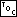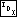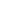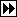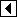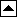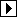The “obvious” way to copy a binary tree is recursive. Here's a basic recursive copy, hard-wired to allocate memory with malloc() for simplicity:

```76. <Recursive copy of BST, take 1 76> =
/* Makes and returns a new copy of tree rooted at x. */
static struct bst_node *bst_copy_recursive_1 (struct bst_node *x) {
struct bst_node *y;

if (x == NULL)
return NULL;

y = malloc (sizeof *y);
if (y == NULL)
return NULL;

y->bst_data = x->bst_data;
return y;
}
```

But, again, it would be nice to rewrite this iteratively, both because the iterative version is likely to be faster and for the sheer mental exercise of it. Recall, from our earlier discussion of inorder traversal, that tail recursion (recursion where a function calls itself as its last action) is easier to convert to iteration than other types. Unfortunately, neither of the recursive calls above are tail-recursive.

Fortunately, we can rewrite it so that it is, if we change the way we allocate data:

```77. <Recursive copy of BST, take 2 77> =
/* Copies tree rooted at x to y, which latter is allocated but not    yet initialized. */
static void bst_copy_recursive_2 (struct bst_node *x, struct bst_node *y) {
y->bst_data = x->bst_data;

if (x->bst_link != NULL)     {
}
else     y->bst_link = NULL;

if (x->bst_link != NULL)     {
}
else     y->bst_link = NULL;
}
```

Exercises:

1. When malloc() returns a null pointer, bst_copy_recursive_1() fails “silently”, that is, without notifying its caller about the error, and the output is a partial copy of the original tree. Without removing the recursion, implement two different ways to propagate such errors upward to the function's caller:

1. Change the function's prototype to:
```    static int bst_robust_copy_recursive_1 (struct bst_node *,                                             struct bst_node **);
```
2. Without changing the function's prototype. (Hint: use a statically declared struct bst_node).

In each case make sure that any allocated memory is safely freed if an allocation error occurs. [answer]

2. bst_copy_recursive_2() is even worse than bst_copy_recursive_1() at handling allocation failure. It actually invokes undefined behavior when an allocation fails. Fix this, changing it to return an int, with nonzero return values indicating success. Be careful not to leak memory. [answer]